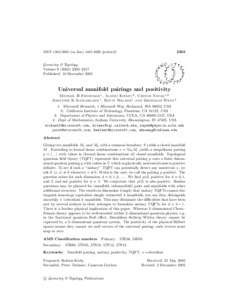# Universal manifold pairings and positivity

Freedman, Michael H. and Kitaev, Alexei and Nayak, Chetan and Slingerland, Joost and Walker, Kevin and Wang, Zhenghan (2005) Universal manifold pairings and positivity. Geometry & Topology, 9. pp. 2303-2317. ISSN 1465-3060Previewmore...Add this article to your Mendeley library

## Abstract

Gluing two manifolds M1 and M2 with a common boundary S yields a closed manifold M. Extending to formal linear combinations x=ΣaiMi yields a sesquilinear pairing p=⟨,⟩ with values in (formal linear combinations of) closed manifolds. Topological quantum field theory (TQFT) represents this universal pairing p onto a finite dimensional quotient pairing q with values in C which in physically motivated cases is positive definite. To see if such a “unitary" TQFT can potentially detect any nontrivial x, we ask if ⟨x,x⟩≠0 whenever x≠0. If this is the case, we call the pairing p positive. The question arises for each dimension d=0,1,2,…. We find p(d) positive for d=0,1, and 2 and not positive for d=4. We conjecture that p(3) is also positive. Similar questions may be phrased for (manifold, submanifold) pairs and manifolds with other additional structure. The results in dimension 4 imply that unitary TQFTs cannot distinguish homotopy equivalent simply connected 4–manifolds, nor can they distinguish smoothly s–cobordant 4–manifolds. This may illuminate the difficulties that have been met by several authors in their attempts to formulate unitary TQFTs for d=3+1. There is a further physical implication of this paper. Whereas 3–dimensional Chern–Simons theory appears to be well-encoded within 2–dimensional quantum physics, e.g. in the fractional quantum Hall effect, Donaldson–Seiberg–Witten theory cannot be captured by a 3–dimensional quantum system. The positivity of the physical Hilbert spaces means they cannot see null vectors of the universal pairing; such vectors must map to zero.

Item Type: Article manifold pairing; unitary; positivity; TQFT; s–cobordism; Faculty of Science and Engineering > Mathematical Physics 10555 https://doi.org/10.2140/gt.2005.9.2303 Dr. Joost Slingerland 21 Feb 2019 15:29 Geometry & Topology Mathematical Sciences Publishers (MSP) YesItem control page